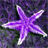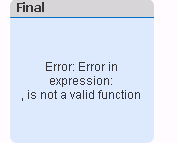# QlikView App Dev

Discussion Board for collaboration related to QlikView App Development.

Announcements
Support Case Portal has moved to Qlik Community! Read the FAQs to start exploring Support resources.
cancel
Showing results for
Did you mean:Creator

## Excluding filters in Set Analysis

Hello All,

i need to exclude multiple filters while calculating the below value

This doesnt work -

vExp_BARWERT=

((sum(\$(vField_Barwert_Angebote))*Avg(Quote/100))*(Sum(\$(vField_Bedarf))/Sum(total {<[Werk Bezeichnung]=,Commodity =,Werk=,[Anfragende Marke]=>}\$(vField_Bedarf))))

This Works-

vExp_BARWERT=

((sum(\$(vField_Barwert_Angebote))*Avg(Quote/100))*(Sum(\$(vField_Bedarf))/Sum(total {<[Werk Bezeichnung]=>}\$(vField_Bedarf))))

How can i exclude multiple filters in the set analysis? i am using \$(vExp_BARWERT) in the chart

Thanks

Supriya

5 RepliesMVP

If second one works, I believe first one may work.

Before develop something, think If placed (The Right information | To the right people | At the Right time | In the Right place | With the Right context)MVP

When you say it doesn't work, what exactly do you mean? You get error? Unexpected result? or what is wrong?Creator
Author

i am trying to calculate yearly Barwert from normal one and Scale it-

vExp_Barwert_Yearly=

(sum(\$(vField_Barwert_Angebote))*Avg(Quote/100))*(Sum(\$(vField_Bedarf))/Sum(total {<[Werk Bezeichnung]=,Commodity =,Werk=,[Anfragende Marke]=>}\$(vField_Bedarf)))

Result -vExpBarwert_Yearly_Total=

(sum(total <Werk,[Werk Bezeichnung],[Anfragende Marke],Commodity> \$(vField_Barwert_Angebote))*Avg(Quote/100))

ResultScalenumber =

if(alt(\$2,\$1)>=1000000000, num(\$1/1000000000, '#.##0,00'),

if(alt(\$2,\$1)>=1000000, num(\$1/1000000, '#.##0,00'),

if(alt(\$2,\$1)>=1000, num(\$1/1000, '#.##0,00'),

num(\$1, '#.##0,00'))))

Final =\$(vScaleNumber(\$(vExp_Barwert_Yearly), \$(vExp_Barwert_Yearly_Total)))but when i remove the multiple filter exclusion and make below equation as -

vExp_Barwert_Yearly=

(sum(\$(vField_Barwert_Angebote))*Avg(Quote/100))*(Sum(\$(vField_Bedarf))/Sum(total {<[Werk Bezeichnung]=>}\$(vField_Bedarf)))something is going wrong with vExp_Barwert_Yearly calculation - is there any other way?

Thanks

SupriyaMVP

So, this is a known issue.. because the delimiter for paramterized variable is comma, the comma you are using in your set analysis is seens as different arguments for the variable. I have seen marcus_sommer‌ provide a solution for this, may be he can provide it yet again here as I don't really remember what he has suggested in the pastMVP & Luminary

Sunny is right and the commas within the total-variable will be treated as parameter-delimiter. I think if you replaced the commas there maybe with '|' and then reversing it again by calling the \$2 within the variable Scalenumber it could be bypassed.

Whereby the approach of such bypassing is quite ugly and error-prone and I wouldn't really suggest them else I would try to avoid these comma-issue completely. One way could be just not using such complex constructs in variables because the aim of the variables is to simplify the developer-life and not the opposite and therefore it could be the easier way just to use normal expressions.

Whereby if I think again a doubled \$-sign expansion might be also working in this case. This meant the following call:

=\$(vScaleNumber(\$(vExp_Barwert_Yearly), \$(=\$(vExp_Barwert_Yearly_Total))))

- MarcusCommunity Browser